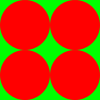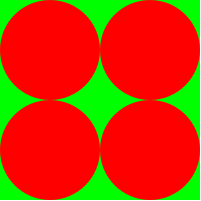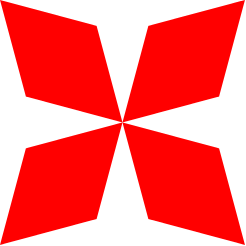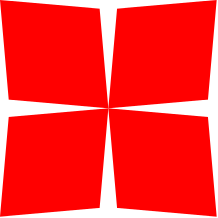Review

• Remember how we write simple and combined expressions of calling functions

(+ 2 3)
(* 2 3 4)
(+ 2 (* 4 5) (* 5 (/ 6 7)))
• and how we define new variables and new functions, and then test or call these functions

(define Pi 3.1415)

;returns area of a circle with given radius

(check-expect (circleArea 10) 314.15)

(circleArea 15)

Review exercise

• Write a function to compute the volume of a sphere $$\frac{4}{3}\pi r^3$$

Working with images

• DrRacket provides a library to work with images: activate teachpack image.ss

• It includes several commands to produce images:

(circle 100 "solid" "red")
(square 100 "solid" "yellow")
(rectangle 50 100 "outline" "green")
(triangle 100 "outline" "black")
(ellipse 50 100 "solid" "purple")
(rhombus
80 ;side length
150 ;one of the angles
"solid" "magenta")
(star 40 "solid" "firebrick")
(empty-scene 100 200)

Image dimensions

• Dimensions in image related functions are expressed in terms of pixels. If the function requires two dimensions the first is horizontal (x-dimension) and the second is vertical (y-dimension)

(rectangle 50 100 "outline" "green")
• An image is within a rectangular containing box, whose dimensions can be measured as follows:

(image-width (circle 100 "solid" "red"))
(image-height (circle 100 "solid" "red"))

Colors

• Commands for drawing shapes take a color parameter.

• A color is a combination of basic colors red-green-blue.

• There are predefined colors like "magenta", "brown", "orange"

• but one can create a new color by combining different amounts of basic colors, where amount is measured on a scale between 0-255, using the command (color ... ... ...):

(circle 100 "solid" (color 170 50 20))

Drawing style of basic shapes

• Commands for drawing basic shapes take a "mode" parameter for drawing style. It can be one of "solid", "outline".

• Instead of "solid" one can also use an integer between 0-255 indicating transparency. Compare the following:

(circle 100 70 (color 0 0 200))
(circle 100 255 (color 0 0 200))

Combining images

• Some basic commands can be used to place images side by side, above or over one another:

(beside (circle 100 70 (color 0 0 200)) (square 250 "solid" "red"))
(above (circle 100 70 (color 0 0 200)) (square 250 "solid" "red"))
(overlay (circle 100 70 (color 0 0 200)) (square 250 "solid" "red"))
(overlay/align "right" "bottom" (circle 100 "solid" "red") (square 250 "solid" "green"))

Exercise

Write a function which takes a size parameter, and two color parameters:

    (define (myShape size color1 color2)
...

(myShape 100 "red" "green")
(myShape 200 "red" "green")

and draws shapes as the follows, whose outer size is equal to the given size:Exercise solution:

    (define (myShape size color1 color2)
(overlay
(above
(beside (circle (/ size 4) "solid" color1) (circle (/ size 4) "solid" color1))
(beside (circle (/ size 4) "solid" color1) (circle (/ size 4) "solid" color1)))
(square size "solid" color2)))
(myShape 100 "red" "green")

Case study: checker board

We wish to build a checker board of given size and colors.

In doing so we utilize two helper functions as below:

        ;Function fullchecker: size:Number color1: String color2:String -> Image
;Returns an 8 by 8 checker board
(define (fullchecker size color1 color2)

;Function checker2: size:Number color1: String color2:String -> Image
; draws a checker board 2 by 2
(define (checker2 size color1 color2)
(above
(beside
(square size "solid" color1)
(square size "solid" color2))
(beside
(square size "solid" color2)
(square size "solid" color1))))

;returns an image which replicates the input image 2 by 2
(above
(beside image image)
(beside image image)))

(fullchecker 40 "red" "green")

Coordinate system and finer placement

• A 2-dimensional coordinate on an image's containing box is expressed in terms of x and y coordinates. Both are measured from top-left corner corresponding to (0, 0) position. x-coordinate increases rightwards, and y-coordinate increases downwards.

• One can control how an image is placed over another using coordinates:

(place-image
(circle 100 70 (color 0 0 200))
50 150  ; x and y coordinates to put the center of the first image on the second
(square 250 "solid" "red"))
• Some commands require two coordinate points:

(add-line
(square 100 "solid" "red") ;on what image we are adding the line
10 50   ;first coordinate, beginning of line
90 70  ;second coordinate, end of line
"green")  ;the line color

Scaling, flipping, rotating, and angles

• Rotation angles are in degrees, counter-clockwise:

(rotate 10 (square 100 "outline" "black"))
(rotate -30 (ellipse 100 20 "outline" "black"))
• Images can be scaled to be smaller or larger, or with different scaling factors for two dimensions:

(scale 2 (ellipse 20 30 "solid" "blue"))
(scale/xy 2 4 (ellipse 20 30 "solid" "blue"))

(overlay
(ellipse 20 40 "solid" "red")
(rotate 90 (scale/xy 2 4 (ellipse 20 30 "solid" "blue"))))
• Images can be flipped horizontally or vertically:

(beside
(rotate 30 (square 50 "solid" "red"))
(flip-horizontal
(rotate 30 (square 50 "solid" "blue"))))

(above
(star 40 "solid" "firebrick")
(scale/xy 1 1/2 (flip-vertical (star 40 "solid" "gray"))))

Exercise

Write a function to draw a butterfly of given size and color:

    (define (butterfly size color angle)
...

(butterfly 200 "red" 60)
(butterfly 200 "red" 80)

which produces an image like follows, whose outer size is equal to the given size:,Exercise solution:

    (define (butterfly size color angle)
(beside
(above
(rotate 45 (rhombus (/ size 2) angle "solid" color))
(rotate -45 (rhombus (/ size 2) angle "solid" color)))
(above
(rotate 315 (rhombus (/ size 2) angle "solid" color))
(rotate -315 (rhombus (/ size 2) angle "solid" color)))))

(butterfly 200 "red" 60)
(butterfly 200 "red" 80)

Animations

• An animation consists of a series of pictures called frames, where each frame $$n$$, is slightly different from the previous one, $$n-1$$, thus creating the effect of a smooth motion.

• DrRacket provides a system for creating animations, when you enable teachpack universe.ss

Animations: creating a frame

• We need a function to create an animation frame. In its simplest form this function can take the frame no:

(define (animation1 frameNo)
(place-image
(circle 20 "solid" "green")
50 frameNo  ;note how the y-coordinate is related to frameNo
(empty-scene 400 400)))

The above function is intended to progress a ball downwards over an empty-scene as frame no increases, thus creating an animation of a falling ball.

Animations: starting

• The Racket function (animate ...) is used, its only parameter is the frame creating function of our choosing:

(animate animation1)

The animation is shown on a separate window, and can be stopped by either closing that window or pressing 'stop'.

Exercise

• Animate two moving balls, one going down and one going horizontally.
• define two constants which determine speeds of balls, i.e. they can be different from 1 pixel/frame.

Exercise solution

    (define (animation2 frameNo)
(place-image
(circle 20 "solid" "red")
frameNo 50
(place-image
(circle 20 "solid" "green")
50 frameNo  ;note how the y-coordinate is related to frameNo
(empty-scene 400 400))))
(animate animation2)

(define speedX 1.2)
(define speedY 0.5)
(define (animation2 frameNo)
(place-image
(circle 20 "solid" "red")
(* speedX frameNo) 50
(place-image
(circle 20 "solid" "green")
50 (* speedY frameNo)  ;note how the y-coordinate is related to frameNo
(empty-scene 400 400))))
(animate animation2)